JieJiSS' Blog 2019-09-15T10:59:15.095Z https://blog.jiejiss.com/ JieJiSS (Pan RZ) Hexo 建立FIKL四个根域名服务器的影响 https://blog.jiejiss.com/建立FIKL四个根域名服务器的影响/ 2019-06-30T01:25:38.000Z 2019-09-15T10:59:15.095Z

• 常用网站DNS解析速度变化不大，DNS递归服务器该走缓存还是走缓存。非常用网站解析可能变快，但幅度有限。
• DNSSEC大失败
• DNS污染触手可及，来自上游的污染使得下游的自建DNS不再稳定，除非放弃FIKL这四个IP
• 与之对应的是老美主动放弃了境内10台DNS根服务器的管辖权（不是镜像服务器哟，是一台主根服务器和九台辅根服务器）
• 老美当然是不可能吃亏的，它放弃这些只能说明这些对它没用
• 不说了，就这样吧
]]>
<blockquote> <p>根据工信部的公告，工信部同意中国互联网络信息中心设立域名根服务器（F、I、K、L 根镜像服务器）及域名根服务器运行机构，负责运行、维护和管理编号分别为 JX0001F、JX0002F、JX0003I、JX0004K、JX0005L、JX0006L

## Bi-LSTM

### RNN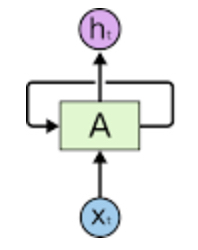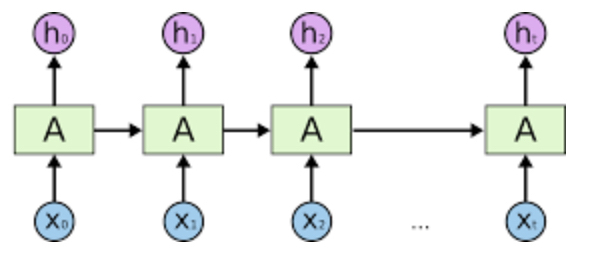BPTT 算法使得梯度可以在时间通道上传递，但是由于局部梯度受过往梯度的累计影响是指数级的（设此时刻到下一时刻的权重为 $$s_t$$，则 Sigmoid 对应的梯度递推公式为 $$\frac{\partial\,{\rm Loss}_t}{\partial\,s_{t-1}^*}=(s_{k-1}*(1-s_{k-1}))*(W^T\times \frac{\partial\,{\rm Loss}_t}{\partial\,s_t^*})$$，显然 Sigmoid 的梯度 $$s_{k-1}*(1-s_{k-1})$$ 会累乘起来，以指数的方式影响结果），所以容易引发梯度爆炸或者梯度消失的问题。解决梯度消失可以换用 ReLU，只不过更容易发生梯度爆炸。梯度爆炸则可以通过设置阈值来避免。另外一种解决的思路就是更改 Cell 的结构，如 LSTM 网络和 GRU 网络。补充知识：LSTM 训练比 GRU 慢，模型更大，但结果更好。GRU 是在对 LSTM 进行简化的基础上产生的，所以一般大家如果要用到 RNN，都会选择直接上 LSTM。

### 双向RNN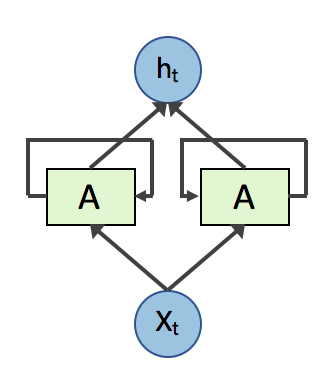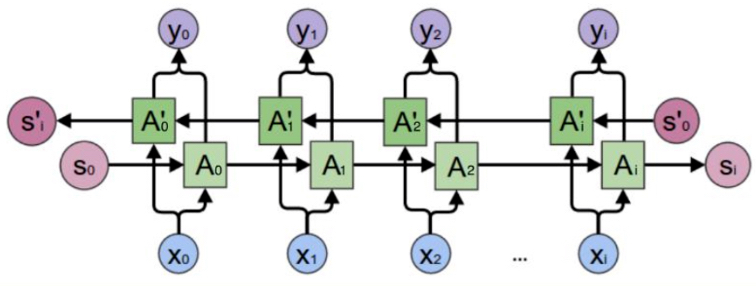### LSTM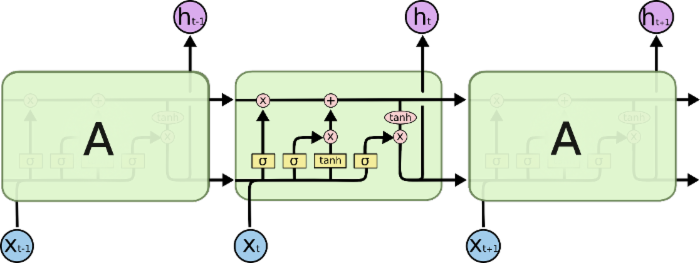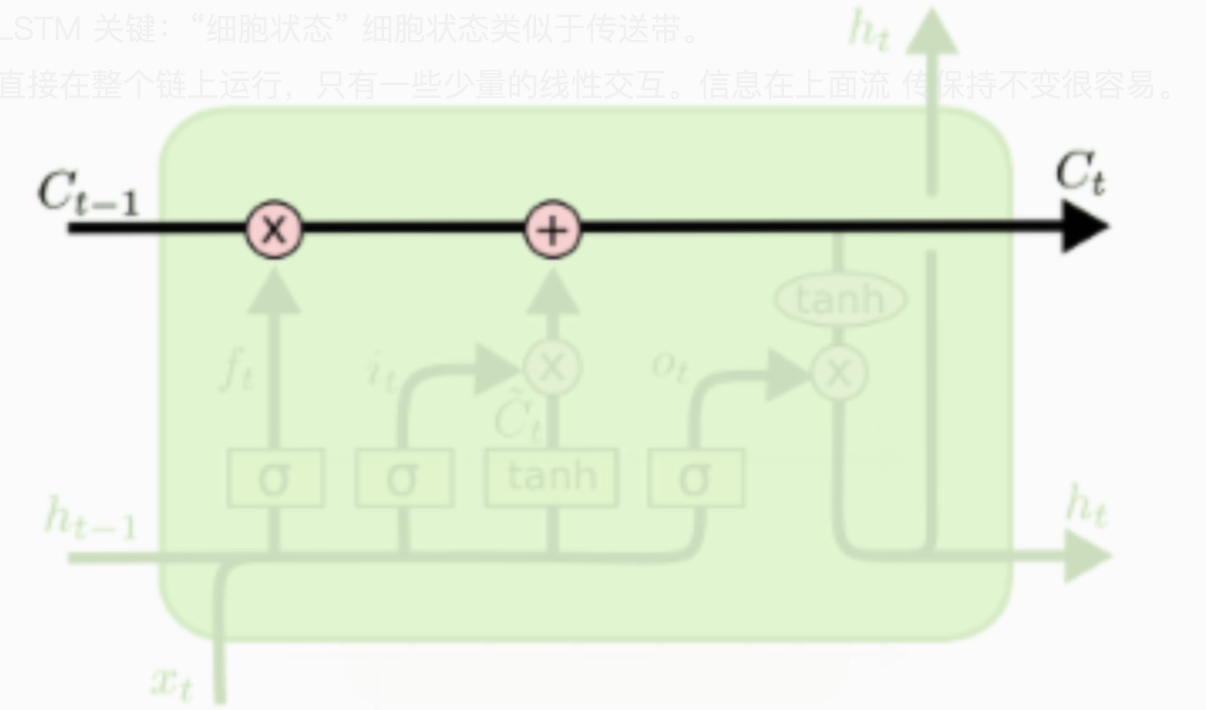#### 遗忘门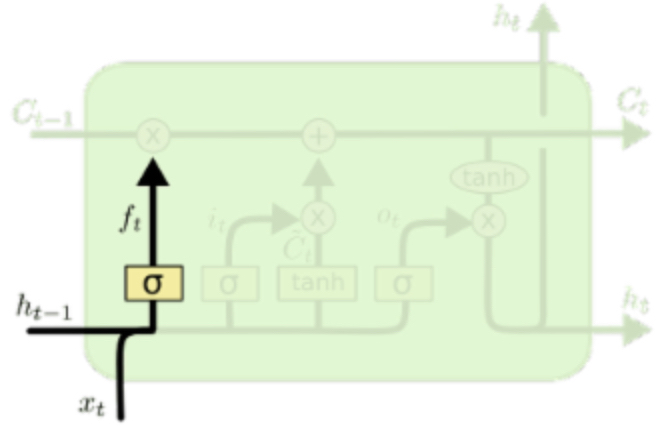$$\sigma$$ 是 Sigmoid 函数。于是很容易发现，上一时刻的 hidden state $$h_{t-1}$$ 与此时刻的输入 $$x_t$$ 级联之后，经过 Sigmoid 函数变换为一个 $$(0, 1)$$ 之间的值并与 $$C_{t-1}$$ 相乘（element wise）。如果 $$\sigma$$ 函数的输出 $$f_t$$ 接近 0，相当于 $$C_{t-1}$$ 这个信息被忘掉了。

#### 输入门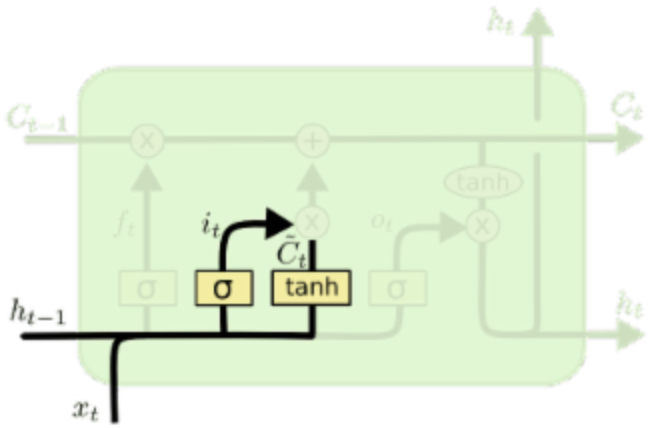#### 输出门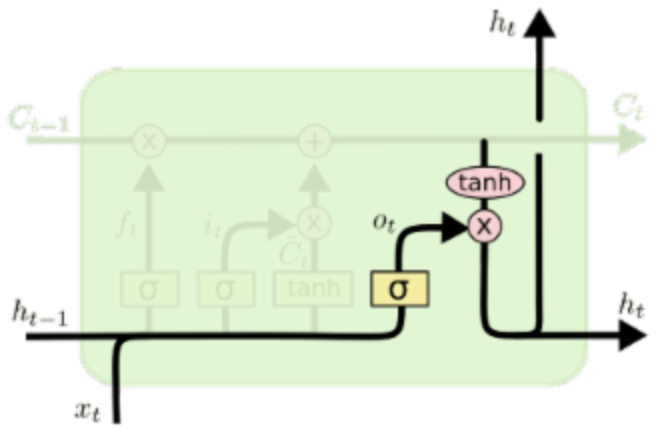## CRF

Linear-CRF 的数学定义：

$$X=(X_1,X_2, \cdots,X_n)$$$$Y=(Y_1,Y_2,\cdots,Y_n)$$ 均为线性链表示的随机变量序列，在给定随机变量序列 $$X$$ 的情况下，随机变量 $$Y$$ 的条件概率分布 $$P(Y|X)$$ 构成条件随机场，也即满足马尔科夫性：

$P(Y_i | X,Y_1,Y_2,\cdots,Y_n)=P(Y_i|X,Y_{i−1},Y_{i+1})$

CRF 层以 Bi-LSTM 层的输出为输入，其可以通过学习数据集中不同 label 间的转移概率从而修正 Bi-LSTM 层的输出。例如，对于 BIO 模型，不存在 I 开头的词，也不存在连续两个 B。如果 Bi-LSTM 给出了上述输出，则 CRF 层可以基于转移概率矩阵对其进行修改。

]]>
<blockquote> <p>主要也是最近刚在学校的某“人工智能研讨会”上展示了一个基于这玩意的项目，趁着还记得原理赶紧写下来。</p> </blockquote> <h2 id="bi-lstm">Bi-LSTM</h2> <p>这玩意全称是双向长短时记忆网络（Bi-dire
JavaScript 位移动运算的奇葩特性 https://blog.jiejiss.com/JavaScript-位移动运算的奇葩特性/ 2019-05-10T00:40:04.000Z 2019-05-10T01:38:46.000Z

p = 180q = -66（分别为大于0和小于0的随机整数）

• Python 2:

• Python 3: The same as Python 2

• php:

• Julia:

• C/C++: There's no need to test

• perl:

• ruby:

• Java: I have no java development env on my Mac

]]>
<blockquote> <p>本文中 <code>a</code> 的取值范围是 <code>[-2147483648, 2147483647]</code>，<code>b</code> 为非负整数</p> </blockquote> <p>是因为对于位移动运算 <code>
A Brand New Way to Hide Important String Constants in JS https://blog.jiejiss.com/A-Brand-New-Way-to-Hide-Important-String-Constants-in-JS/ 2019-05-08T04:20:25.000Z 2019-09-15T10:27:57.259Z

This algorithm is designed & implemented by JieJiSS.

Anyway, 你说英文写博客好，那我就试试呗

## 0x00 Basic Variables & Helper Functions

Define raw: The variable raw is used to represent the raw string constant which needs to be encoded. Beware that this is not an encryption algorithm, which means that it is not cryptographically secure.

### 0x00.1 VariabledataArr: number[]

This is a pre-generated array which stores at least 9 (1+2+2*2+2) elements.

Define $$m$$ as: $m = \max\,(2, \lceil\frac{\texttt{raw.length}}{7}\rceil)$

Magic number 7: Every ASCII string that has no more than 7 characters can be encoded to a base64 string that has a maximum length of 10 characters (exclude trailing equal signs), which can be safely treated as a 36-decimal integer without any precision lost under the IEEE754 double data type. That's because in that case, parseInt(encodedStr, 36) always returns an integer that is lower than Number.MAX_SAFE_INTEGER, i.e. $$2^{53}-1=9007199254740991$$, since 9007199254740991 .toString(36) returns "2gosa7pa2gv" (which has 11 characters).

### 0x00.2 Helperf(x)

This helper function is used to calculate the "system" of the x-th element of dataArr. f(x) could be any function which satisfied following criteria:

1. $$f(0) \equiv 10 \mod 256$$
2. $$f(1) \equiv 36 \mod 256$$
3. $$f(2) \equiv 2 \mod 256$$
4. $$f(3) \equiv 2 \mod 256$$
5. $$\lfloor f(k) \rfloor =f(k) \quad(k\in\mathbb N^*)$$
6. $$f(k)>0\quad (k=0,1,2,3)$$

Here are some available f(x)s: \begin{aligned}f_1(x)&=-27x^3+179x^2-126x+10\\f_2(x)&=101x^3-333x^2+258x+266\end{aligned}

How to create a valid $$f(x)$$?

Let $$f(x)=ax^3+bx^2+cx+d$$.

According to the first criterion, we know $$f(0)=d \equiv 10 \mod 256$$.

So, we can simply choose values that look good for $$d$$: 10, 266, 522, …

For similar reasons, we know that $$f(1)-d=a+b+c\equiv 26\mod 256$$, $$f(2)-d=8a+4b+2c\equiv 248\mod 256$$, and $$f(3)-d=27a+9b+3c \equiv 248\mod 256$$. Note that $$27a+9b+3c\equiv 0\mod 3$$, thus in order to get integer values for $$a$$, $$b$$, and $$c$$, we need to ensure that $$27a+9b+3c=248+(3k+1)\times256$$. Detailed explanation is that $$248=3\times82+2$$ and $$256=3\times85+1$$, hence $$248+256+3k\times256 = 504+3k\times256$$ can always be divided by 3. After these calculations, we can simply solve the ternary systems of equations and get a possible combination for $$a$$, $$b$$ and $$c$$.

To quickly solve such systems of equations, you may need to know how to use the Gaussian Elimination.

Cited from https://yutsumura.com/solving-a-system-of-linear-equations-using-gaussian-elimination/: \begin{align*} x+2y+3z &=4 \\ 5x+6y+7z &=8\\ 9x+10y+11z &=12 \end{align*} The three elementary row operations on a matrix are defined as follows.

1. Interchanging two rows: $$R_i \leftrightarrow R_j$$ interchanges rows $$i$$ and $$j$$.

2. Multiplying a row by a non-zero scalar (a number):

$$t R_i$$ multiplies row ii by the non-zero scalar (number) $$t$$.

3. Adding a multiple of one row to another row:

$$R_j+tR_i$$ adds $$t$$ times row $$i$$ to row $$j$$.

Solution

First, the augmented matrix of the system is: $A=\left[ \begin{array}{rrr|r} 1 & 2 & 3 & 4 \\ 5 & 6 & 7 & 8 \\ 9 & 10 & 11 & 12 \end{array} \right].$ We apply elementary row operations as follows to reduce the system to row echelon form. $A \xrightarrow{R_3 -9R_1} \left[\begin{array}{rrr|r} 1 & 2 & 3 & 4 \\ 5 & 6 & 7 & 8 \\ 0 & -8 & -16 & -24 \end{array}\right]\xrightarrow{-\frac{1}{8}R_3} \left[\begin{array}{rrr|r} 1 & 2 & 3 & 4 \\ 5 & 6 & 7 & 8 \\ 0 & 1 & 2 & 3 \end{array}\right]$

$\xrightarrow{R_2-5R_1} \left[\begin{array}{rrr|r} 1 & 2 & 3 & 4 \\ 0 & -4 & -8 & -12 \\ 0 & 1 & 2 & 3 \end{array}\right]\xrightarrow{-\frac{1}{4} R_2} \left[\begin{array}{rrr|r} 1 & 2 & 3 & 4 \\ 0 & 1 & 2 & 3 \\ 0 & 1 & 2 & 3 \end{array}\right]$

$\xrightarrow{R_3-R_2} \left[\begin{array}{rrr|r} 1 & 2 & 3 & 4 \\ 0 & 1 & 2 & 3 \\ 0 & 0 & 0 & 0 \end{array}\right]$

The last matrix is in row echelon form.

The corresponding system of linear equations of it is: \begin{align*} x+2y+3z &=4\\ y+2z&=3 \\ 0z&=0 \end{align*}

### 0x00.3 Helperb64(str)

This helper function encodes str to a modified-base64 string literal. Note that it should remove all trailing equal signs. Also, note that the whole b64templ string except its last three characters is reversed to annoy browsers' built-in atob base64 decode function.

### 0x00.4 Helperdeb64(str)

Decode base64 encoded str.

### 0x00.5 HelperrndChar()

This helper function is used to generate a random character ranged in a-zA-Z0-9.

### 0x00.6 HelperpadLeft(str, len, ch)

This helper function will repeatedly add ch as left padding to str until str.length === len.

## 0x01 Generator

For instance, we'd like to hide a string constant "Hello, World!" from users.

Step 1. Slice this string constant into $$m$$ pieces:

Now, we have an array sliced: string[] which contains sliced parts of the string constant:

Step 2. Record the letter cases of all characters in sliced: string[]. Also, in this step we will need to encode the lowercase of each string element's of sliced using the b64() helper function, and store it in partial: string[].

In this case, rb64encodedText = ["r3oRi3BR19", "oHALi3tc"][i].

Now, cases becomes [76, 112] ([0b1001100, 0b1110000], was once [0b0001001100, 0b01110000]), and partial becomes["r3ori3br19", "ohali3tc"]. You may have noticed that the 0s before the first 1 won't affect the result, so we'll use the padLeft helper function to retrieve those missing 0s later when we're attempting to decode it.

Step 3. Replace all +/= in partial[i] to random characters ranged in a-zA-Z0-9, and store relative information in special: number[]. This step makes sure that parseInt(partial[i], 36) can always return a valid integer (stored inside magicNums: number[]).

According to Base64 - Wikipedia, equal signs only appear at the end of a base64 encoded string, so there's no need to deal with it because we have trimmed trailing equal signs in former steps.

Note that on line 4, we give chunks: string[] an initial value ["0", "0"]. That's because parseInt("") returns NaN, and in most cases base64Text won't include "+" or "/", so chunks will likely to remain unmodified. Hence, we need to make sure parseInt(chunks[i]) will not return NaN.

Now, special becomes [0,0,0,0] since there's no + or / presents in base64-encoded text, and magicNums becomes [2752522766809245, 1918386016464].

Step 4. Concat magicNums.length, magicNums, special and cases together, and store it as dataArr. Then, convert dataArr to data.

I've recognized that symbols like + and / rarely appears in the base64 encode method, so there'll always be a huge amount of 0s in special: number[]. Thus, if we convert all the 0s in dataArr to "", we can save quite a lot of bytes.

Conclusion for Generator Part

Summary all codes together:

And the input is encoded as:

## 0x02 Decoder

Assume that we have fetched the encoded data from our backend:

Our backend codes:

Our frontend codes:

And now, we need to apply several operations to the encoded variable in order to decode it.

First of all, we will convert encoded: string to an array named firstStepArr:

And then, we'll try slice this array into 3 parts. Since the encoded str contains three parts, magicNums, special and cases, we can have a glance at previous codes:

As we can see, let $$l=\texttt{sliced.length}$$, then we have $$\texttt{partial.length}=l$$ and $$\texttt{cases.length}=l$$. Also, we can infer that $$\texttt{special.length}=2l$$ and $$\texttt{magicNums.length}=l$$. Thus, we can easily know that $$\texttt{firstStepArr.length}=4l+1$$.

So, we can declare a size: number variable as we will need to use it to slice the firstStepArr and generate a secondStepArr: number[][] later:

In the third step, we try to convert numbers to decoded strings. The $$f(x)$$ function mentioned above now shows its necessity:

Yep, we now have the raw text decoded as thirdStepArr!

Conclusion for Decoder Part

Final codes:

The decoded result:

## 0x03 Use Without Decoder

Well, you can encode users' input and compare it with the already encoded text. However, you will have to modify rndChar to achieve that, since in some rarely seen cases it will cause different encoded result for the same input text.

My suggestion is that you can use a controllable PRNG (Pseudo Random Number Generator) to replace Math.random(), for example:

Don't forget to call resetAll() to reset exists to [seed % modulo] and index to -1 every time you want to encrypt a string!

]]>
<blockquote> <p>This algorithm is designed &amp; implemented by JieJiSS.</p> <p>这篇博客应该会是全英文的（除了这几句话以外）……一方面是为了学校安排的几周后的“高中生人工智能教育”研讨会上的全英文 P
LiveRe评论在hexo-theme-even下偶发消失问题 https://blog.jiejiss.com/LiveRe评论在hexo-theme-even下偶发消失问题/ 2019-05-05T05:52:17.000Z 2019-05-09T03:56:10.000Z

TL;DR 锅是PJAX的，可以通过修改 _config.yml 修复。

（此处省略在后端 node_modules 中遨游 5 分钟）

（什么？你问我为什么找的这么快？啧，编辑器的文件夹全局搜索功能不就是拿来干这个的嘛（逃

ok，于是显然问题就出在这了。

（此处省略打log调试 3 分钟）

_config.yml 中，每个链接的最后都加上 / 就好了，这样既不用改库（上次抓 Bug 一路抓到 jQuery 里去真是累死我了，详见 ahonn/hexo-theme-even#241 中的 Outdated 的 Code Review），也不用改 LiveRe（这个大概会更累）。

]]>
<blockquote> <p>刚刚遇到的，还热乎着呢</p> </blockquote> <p><strong>TL;DR 锅是PJAX的，可以通过修改 <code>_config.yml</code> 修复。</strong></p> <p>LiveRe评论框是静态博客的福音

1. 相关系数 $$r$$ 值检验

$$a=\hat a, b=\hat b$$\begin{aligned}\text{LHS}&=\sum^n_{i=1}(y_i-\bar y)^2-\frac{\left(\sum^n_{i=1}(x_i-\bar x)(y_i-\bar y)\right)^2}{\sum^n_{i=1}(x_i-\bar x)^2}\end{aligned} 又因为残差一定大于等于 0，所以 $\sum^n_{i=1}(y_i-\bar y)^2\ge\frac{\left(\sum^n_{i=1}(x_i-\bar x)(y_i-\bar y)\right)^2}{\sum^n_{i=1}(x_i-\bar x)^2}$ 所以 $1\ge\frac{\left(\sum^n_{i=1}(x_i-\bar x)(y_i-\bar y)\right)^2}{\sum^n_{i=1}(x_i-\bar x)^2\cdot\sum^n_{i=1}(y_i-\bar y)^2}$$r^2=\frac{\left(\sum^n_{i=1}(x_i-\bar x)(y_i-\bar y)\right)^2}{\sum^n_{i=1}(x_i-\bar x)^2\cdot\sum^n_{i=1}(y_i-\bar y)^2}$ 则有 $$r$$ 为相关系数（查表可得），与自由度 $$n-2$$$$n$$ 为数据总量）有关。$$r$$ 越接近 1 表示两个变量越相关。 $r=\frac{\sum^n_{i=1}(x_i-\bar x)(y_i-\bar y)}{\sqrt{\sum^n_{i=1}(x_i-\bar x)^2\cdot\sum^n_{i=1}(y_i-\bar y)^2}}$ 如果 $$r \ge r_{0.05}$$，则认为有 95% 的概率 $$x$$$$y$$ 是相关的，该线性回归方程有效。否则认为该方程无意义。

1. $$p$$ 值检验：

R 语言：

Excel 中计算 $$p$$ 值的方法的等价 MATLAB 代码：

（可以在这里在线运行 MATLAB 代码）

Citation:

http://blog.sciencenet.cn/home.php?mod=space&uid=651374&do=blog&id=1053456&from=space

https://stattrek.com/probability-distributions/t-distribution.aspx

https://stattrek.com/regression/slope-test.aspx

https://blog.csdn.net/renwudao24/article/details/44465407

]]>
<p>拟合函数为 <span class="math inline">$$y=\hat b x+\hat a$$</span>。使用最小二乘法求 <span class="math inline">$$\hat a$$</span>、<span class="math inlin
JavaScript中Global-Flag RegExp匹配同样字符串结果不同问题 https://blog.jiejiss.com/JavaScript中Global-Flag-RegExp匹配同样字符串结果不同问题/ 2019-05-04T10:32:31.000Z 2019-05-09T03:56:10.000Z 0x00 TL;DR

## 0x01 问题详述

node 版本 10.15.3（LTS）复现成功。

## 0x02 解释

【此处省略面向搜索引擎编程 5 分钟】

（我知道在 replacer 函数里修改外层 RegExp 对象可能会破坏优化……但是没办法，需求所迫啊（逃

]]>
<h2 id="x00-tldr">0x00 TL;DR</h2> <p>是因为对于有 <code>g</code> flag 的正则表达式对象，<code>RegExp#lastIndex</code> 的值会随着匹配而改变。</p> <h2 id="x01-问题详述">0x0

## 0x01 制备原材料

1. WS2812B 全彩 8*32 可编程像素软屏

这个淘宝直接买啦，简单省事

2. ESP8266 WIFI 模块 CP2102 ESP-12E

这个家里有，嘻嘻

3. 光栅

是为了把圆光点变成方形的，看上去更帅气

直接 3D 打印

4. 长期可访问的网络节点

考虑到我有台服务器，就直接用它好了。也可以用有公网 IP 的电脑来做。

5. 杂项：杜邦线，micro USB线，热熔胶枪……

## 0x02 配置服务器端

### 0x02.2 配置反向代理和 HTTP Basic Authentication

（关于 HTTP Basic Authentication 的安全性问题，可以看：Is basic access authentication secure?

]]>
<h2 id="x00-什么是-awtrix">0x00 什么是 awtrix</h2> <p>入坑其实是因为看到了 V2EX 上的一个帖子：<a href="https://www.v2ex.com/t/559292" target="_blank" rel="noopener

]]>
JS定义可变参数函数 https://blog.jiejiss.com/JS定义可变参数函数/ 2019-04-24T08:08:59.000Z 2019-11-06T12:19:48.355Z

↑ 这句话具有极强的筛选作用，这样知道我在说啥的就会关掉这个页面而不会浪费人生中宝贵的 10 分钟

• 多层闭包
• applythis
• ES6 Symbol

## 0x01 Explanation

### 0x01.1 invalidArgumentsHandler

invalidArgumentsHandler 是当传入的函数参数类型和值与已定义的无法匹配时将会调用的函数。它将抛出一个 ReferenceError

### 0x01.2 getType

obj.constructor.name.toLowerCase() 可以获得 obj 的类名。如果 obj 没有 constructor 属性，那么就使用通用的 toString 方法来获得类名。

（进阶内容）注意，尽管用户可能使用 ES6 中引入的 Symbol 来影响 Object.prototype.toString.call(obj) 的结果：

### 0x01.3 defineFlexMethod

#### 传入参数检测

• 如果未指明 argType 参数，则将 argType 初始化为长度为 func.length 且每项均为 "any" 的数组
• 第三个参数 func 必须是函数
• 如果传入了 argType，则必须是个数组，其长度必须等于 func.length

#### 旧值检测

• 如果 obj 对象已经有了 attr 属性，则其必须是函数（也即“方法”）。
• 如果 obj 对象已经有了 attr 方法，且该方法有 __count 属性，则此属性必须是数字。
• 如果 obj 对象已经有了 attr 方法，但该方法没有 __count 属性，则初始化此属性为 0。
• 如果 obj 对象没有 attr 属性，则初始化其为 invalidArgumentsHandler

#### 使用新方法

• 调用该方法时，判断得到的参数的类型和数量。
• 如果和用户最后一次定义时提供的 func 的参数类型和数量匹配，则指定 this 为运行时 this 并使用得到的参数调用 func（注意 apply 的使用）。
• 否则，调用 prevMethodprevMethod 即为上一轮包装的新方法，它也将进行同样的判断。
• 以此类推，直到有某一轮定义的 func 的参数类型和数量与给出的参数类型和数量匹配为止。
• 如果所有的 func 都无法与给出的参数类型和数量匹配，则会最终调用 invalidArgumentsHandler，抛出异常。
]]>
<blockquote> <p>这个法子是 jQuery 之父发明的</p> <p>↑ 这句话具有极强的筛选作用，这样知道我在说啥的就会关掉这个页面而不会浪费人生中宝贵的 10 分钟</p> <p>本文涉及知识点：</p> <ul> <li>多层闭包</li> <li><code
JS实现Trie树 https://blog.jiejiss.com/JS实现Trie树/ 2019-04-23T07:48:48.000Z 2019-05-09T03:56:10.000Z 0x00 写在最前

Trie 树，又名字典树、前缀树，其名称来源于 retrieve 一词（v. 检索），是一种有序树，也属于确定有限自动状态机。其常被用于提供搜索提示。

## 0x01 实现

]]>
<h2 id="x00-写在最前">0x00 写在最前</h2> <blockquote> <p>嗯……这个 Trie 树完全是手撸的，没参考啥教程/博客，所以接口名称和算法实现上<em>可能</em>和常规方法有些许差异。不过功能都是完备且 ok 的（</p> </blockq

「尊前拟把归期说，未语春容先惨咽」xgPby/3QhI0h。（原文：Hello, world!）

## 0x00 作者介绍

「谷雨解字」是类似阿里 Iconfont-webfont 的在线工具。区别是「谷雨解字」提供了字形和编码混淆的功能，即不单提供了中文字体子集化的功能，还可以自己定义字形的映射。比如可以把“甲”字的编码映射到“乙”字的字形上，网页如果加载了该字体，那么网页中出现的所有“甲”字在用户看来就是“乙”字的样子。

「谷雨解字」的用途 - 网页加密、内容混淆 - 网页内容防拷贝 - 网站反爬虫 - SEO （搜索引擎优化） - 敏感词过滤

「寒蝉」是「谷雨解字」配套的 Chrome 浏览器扩展程序。安装该浏览器插件后，可以将网页中的特殊文本用科学字体来渲染。

• 安装教程

## 0x02 玩一玩

「金印累累佩陆离，河梁更赋断肠诗。莫拥旌旗真个去。何处？玉堂元自要论思。且约风流三学士，同醉。春风看试几枪旗。从此酒酣明月夜。耳热。那边应是说侬时。红日已高三丈透，金炉次第添香兽，红锦地衣随步皱」RjKdh/2BCNgo。

## 0x03 优点和不足

### 0x03.2 缺点

]]>
<blockquote> <p>原贴：https://www.v2ex.com/t/553248</p> <p>加密效果样例：</p> <p>「尊前拟把归期说，未语春容先惨咽」xgPby/3QhI0h。（原文：Hello, world!）</p> </blockquote> <h

]]>

## 0x03 正态分布

$$\mu=0,\,\sigma=1$$ 时为标准正态分布： \begin{aligned}f(x)=\frac1{\sqrt{2\pi}}\cdot\exp\left(-\frac{x^2}{2}\right)\end{aligned} 此时 \begin{aligned}\Phi(x)=\frac1{\sqrt{2\pi}}\int_{-\infty}^xe^{-\frac{t^2}2}\,\text{d}t\end{aligned}

### 0x03.1 正态分布的一些性质

#### 在一定区间内取值的概率

$$(\mu-\sigma, \mu+\sigma)\approx68.27\%$$

$$(\mu-2\sigma, \mu+2\sigma)\approx95.44\%$$

$$(\mu-3\sigma, \mu+3\sigma)\approx99.73\%$$

$$(\mu-4\sigma, \mu+4\sigma)\approx99.99\%$$

（长尾理论）

#### 运算性质

\begin{aligned}D(aX+bY)&=a^2D(X)+b^2D(Y)+2ab\operatorname{cov}(X,Y) \\ \Rightarrow D(aX+b)&=a^2D(X)+b^2D(1)+2ab\operatorname{cov}(X,1) \\ &=a^2D(X)+b^2\cdot0+2ab\cdot 0 \\ &=a^2D(X) \end{aligned}

$$\operatorname{cov}(X,Y)$$$$X$$$$Y$$ 的协方差：

\begin{aligned}\operatorname{cov}(X,Y)&=E((X-E(X))(Y-E(Y)))\\&=E(X\cdot Y)-E(X)E(Y)\end{aligned}

$$X$$$$Y$$ 独立时，$$\operatorname{cov}(X,Y) = 0$$

Citation:

]]>
<blockquote> <p>离散型随机变量的数字特征</p> <p>连续型随机变量的数字特征</p> <p>数学期望：<span class="math inline">$$E(X)=\sum_{i=1}^n x_ip_i$$</span></p> <p>方差：<span c
Python的round函数和JS中的Math.round的不同之处 https://blog.jiejiss.com/Python的round函数和JS中的Math-round的不同之处/ 2019-04-03T02:31:48.000Z 2019-05-09T03:56:10.000Z

Citation:

## 具体分析

Python 2 中的 round 采用四舍五入，而 Pyhton 3 中的 round 采用的是大学物理使用的四舍六入五成双（后文会详细解释）。

Python 把 -1.6-1.4 round 到 -2-1，而 JS 则将二者 round 到 -1-2。这是因为 Python 会往绝对值更大的方向“五入”、绝对值更小的方向“四舍”，而 JS 则是往值更大的方向“五入”、值更小的方向“四舍”。

Python 的 round 接受 1 至 2 个参数，而 JavaScript 的 Math.round 只接受一个参数。所以 Python 可以指定舍入精度，而 JS 则只能舍入到整数。

1.15.toFixed(100) 得到了

1.1499999999999999111821580299874767661094665527343750000000000000000000000000000000000000000000000000

JS 和 Python 的 round 都可以正确处理布尔值。然而其内在实现机理是不同的：

• JS 尝试使用 Number 函数将输入转为数字，再对其进行常规的 round 操作。
• Python 尝试调用输入的值的 __round__ 方法，并返回其结果。由于字符串（str 类）没有定义 __round__ 方法，所以无法被 round 函数处理。

“坑 3” 提到的浮点数精度丢失并不能解释 Test Case 9 的结果，因为 Python 和 JS 在底层都使用 IEEE 754 双精度浮点数来表示小数，二者在小数表示上精度相同，但是 Test Case 9 的 Python 和 JavaScript 结果不同。

*当要保留__小数点后 $$n$$ 位__时，*

• 如果第 $$n+1$$ 位不为 5，按照四舍五入规则舍入。
• 如果第 $$n+1$$ 位为 5，但是其后仍有有效数字，则进位。
• 如果第 $$n+1$$ 位为 5，且其后没有任何有效数字：
• $$n$$ 位为奇数时，进位；
• $$n$$ 位为偶数时，舍去。

1.25 保留小数点后一位时，小数点后第三位为 0，第二位为 5，第一位为偶数，所以舍去第二位的 5，第一位保持不变。

JavaScript 的 round 则是简单的四舍五入，没有这么多门道。

]]>
<blockquote> <p>如无特殊说明，本文中的 <code>Python</code> 均指代 <code>Python 3</code>。</p> <p><strong>Citation:</strong></p> <ol type="1"> <li><a href="## 0x01 读出阻值## 0x02 读误差

（灰比银颜色要更深一些，银色更贴近白色）

## 0x03 结语

]]>
<h2 id="x00-辨别方向">0x00 辨别方向</h2> <p>理论上讲，五色环蓝电阻的五个环会呈现出前 4 后 1 的排布：</p> <p><img src="https://i.loli.net/2019/03/29/5c9d798e0c703.jpg" alt="基

## 0x00 妈见打方法一

• 例 1.

这种代码一看就能缩减掉很多行：

• 例 2.

本段似乎使用了只能在 Mac/iOS/Android 系统上查看的 Emoji 表情符号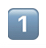，在 Windows 上可能显示为空心方框。

这里需要注意，"①".length === 1"1️⃣".length === 3

长度为 3 是因为 "1️⃣" === "1" + "\ufe0f" + "\u20e3"

稍微高端点的写法：（还是很复杂）

简单点：

但是，如果真的想最好地支持 Unicode，其实应该这么写：

• 例 3.

我数学就是差，怎么地吧！

稍微优化一下：

第 2 行有点长，加个位运算来向下取整：

试试还能不能再短点：

呵呵，其实已经有点优化过头了，就此打住吧。

## 0x01 妈见打方法二

• 例 1.

正常的写法：

其实不是完全等价的写法，因为最上面那个函数只能处理到 $$[- 2^{31}, 2^{31})$$

• 例 2.

这特么是什么鬼？？？

正常的写法：

## 0x02 妈见打方法三

1. 用 TypeScript 写一段面向对象、Promise和async/await还有yield混用的代码，转译成 ECMAScript 7
2. 再用 Babel 转译成 ECMAScript 6
3. 接着用 npm: browserify 或者是 gh: nathan/pax，打包成能直接在浏览器里运行的代码
4. Google Closure Compiler 来压缩代码，选择压缩等级为 SIMPLE_OPTIMIZATIONS
5. Prettier.io 美化回来

## 0x03 妈见打方法四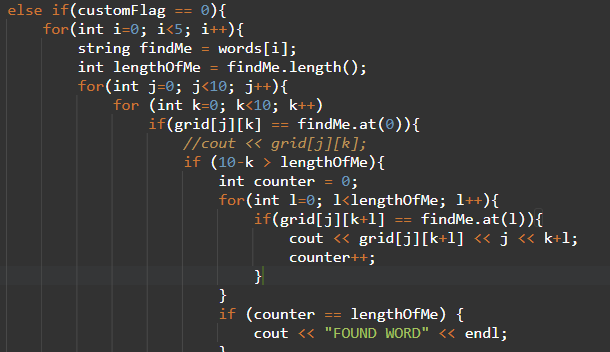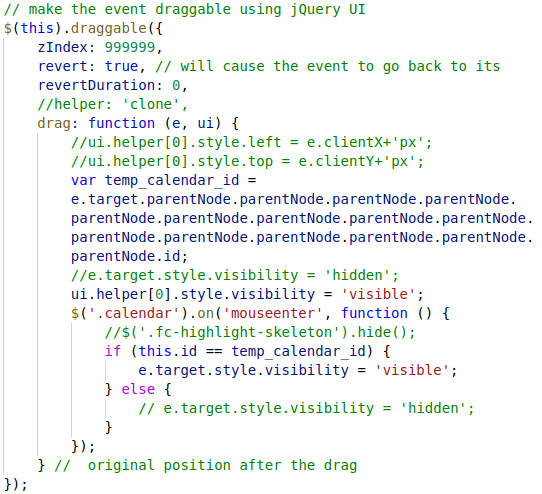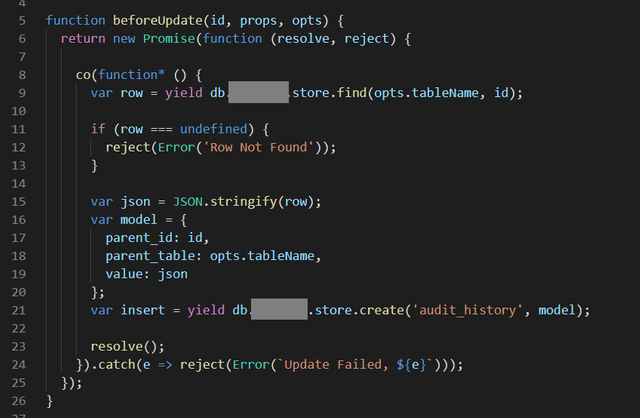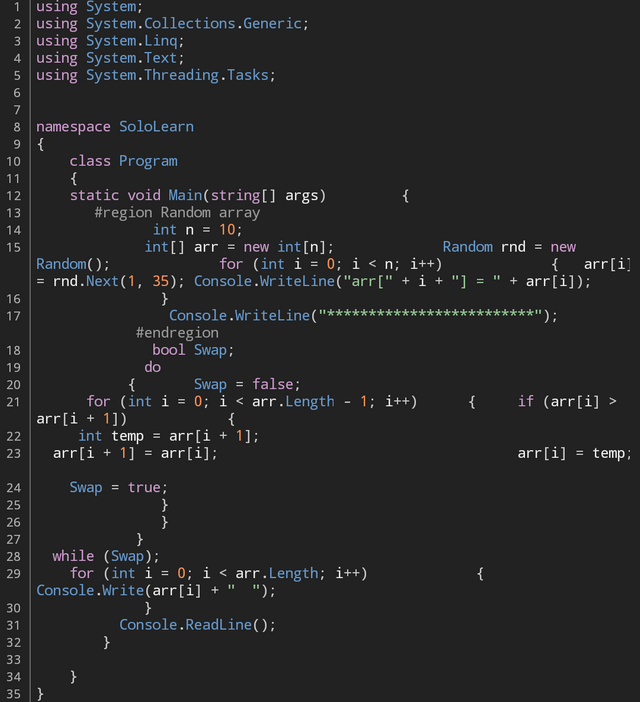]]>
<blockquote> <p>本文不追求列举全部妈见打方法。</p> </blockquote> <h2 id="x00-妈见打方法一">0x00 妈见打方法一</h2> <p>代码写的巨冗杂繁琐。</p> <ul> <li><p>例 1.</p> <figure class=

## 0x02 实现

$${\rm Yang}(m,n)=C_{m-1}^{\,n-1}$$

That's it!

$$$$: 百度百科$$.$$ 杨辉三角

$$$$: 百度百科$$.$$ 二项式定理

]]>
<blockquote> <p>警告：理解本博文要求有一定的数学功底</p> </blockquote> <h2 id="x00-背景">0x00 背景</h2> <p>杨辉三角是一个历史非常悠久（接近 1000 年前发现<span class="math inline">\(^
Contribution In 2018 https://blog.jiejiss.com/Contribution-In-2018/ 2019-03-13T08:39:32.000Z 2019-05-09T03:56:10.000Z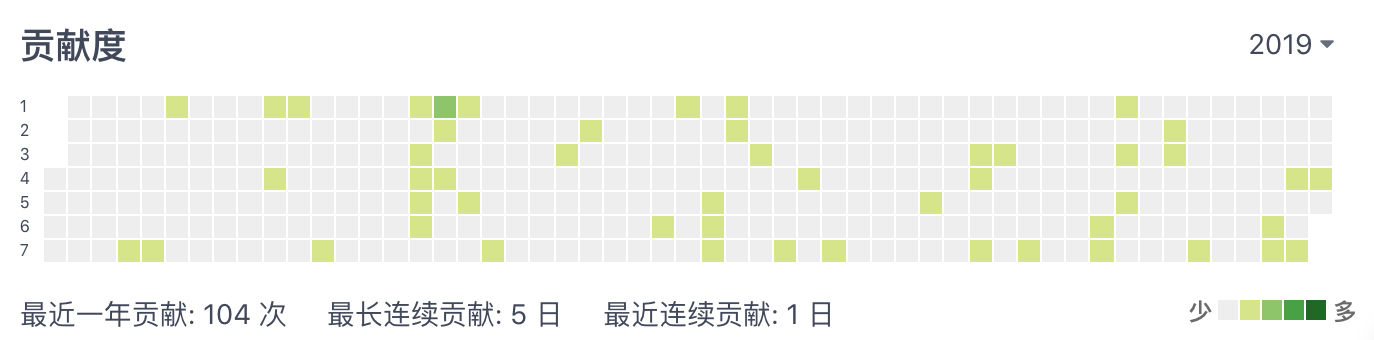（不是同一天截的图）

]]>
<p><img src="https://i.loli.net/2019/03/08/5c81edd753a45.jpg" alt="2018 Contribution Gitee.jpg" title="2018 Contribution Gitee.jpg" width="6